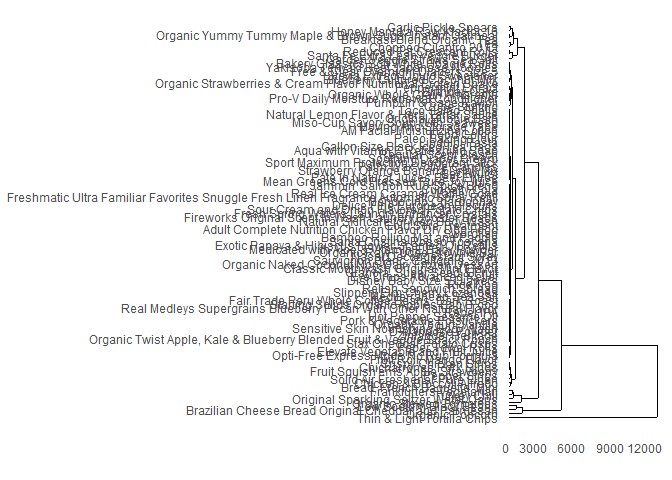Today practice session is about customer segmentation, which has become an essential part of marketing. During the practice we will deal with the RFM model and the heuristic approach, while later on we will demonstrate the automatic segmentation discovery via kmeans and hierarchical clustering.

## Packages

For this practice session you will need next packages:

library(dplyr)
library(ggplot2)
library(gridExtra)
library(data.table)
library(tm) # package for text mining
library(wordcloud) # for word visualization
library(ape) # dendrogram plotting
library(ggdendro) # dendrogram plotting

In case some packages can not be found, place install them with the next command:

install.packages(“name-of-the-package”)

## RFM

Let’s first load a dataset, where there is an information about clients and their orders along with the order details:

orders <- read.table(file.choose(), header=TRUE, sep=',') # orders_rfm.csv

The next logical step is to investigate what kind of data you have.

View(orders)

Let’s perform RFM analysis, which means to calculate three key measures:

1. R - recency score
2. F - frequency score
3. M - monetary score

In our case recency will be expressed as number of days since the last order, frequency will be defined as the number of purchased items, and monetary score is the total amount spent during the defined period. There are a lot of variations of these definitions. For example, when necessary, you may want to aggregate recency component on a yearly basis rather than using days; frequency and monetary scores can be expressed as the percentage of one period to another, etc. Moreover, it is important to define the period under investigation:

First let’s pick date of interest:

reporting_date <- as.Date('2017-03-10', format='%Y-%m-%d')
reporting_date
##  "2017-03-10"

Next, we have to change the type of the date in our table from factor to date:

str(orders)
## 'data.frame':    4378 obs. of  5 variables:
##  $order_id : int 1 1 1 1 2 2 2 2 3 3 ... ##$ product    : Factor w/ 3 levels "a","b","c": 1 2 3 2 1 3 2 1 1 3 ...
##  $client_id : int 473 473 473 473 282 282 282 282 220 220 ... ##$ order_date : Factor w/ 100 levels "2017-01-02","2017-01-03",..: 91 91 91 91 47 47 47 47 55 55 ...
##  $money_spent: num 14.8 112.47 235.69 28.38 8.36 ... orders$order_date <- as.Date(orders$order_date, format='%Y-%m-%d') str(orders) ## 'data.frame': 4378 obs. of 5 variables: ##$ order_id   : int  1 1 1 1 2 2 2 2 3 3 ...
##  $product : Factor w/ 3 levels "a","b","c": 1 2 3 2 1 3 2 1 1 3 ... ##$ client_id  : int  473 473 473 473 282 282 282 282 220 220 ...
##  $order_date : Date, format: "2017-04-02" "2017-04-02" ... ##$ money_spent: num  14.8 112.47 235.69 28.38 8.36 ...

For more details about the format of the dates refer to: https://www.statmethods.net/input/dates.html

Since we are interested only in orders that happened before specified date, we will filter unneccessary orders:

orders <- filter(orders, order_date <= reporting_date)

As we discussed previously, the descriptive part helps to get sense of the data:

length(unique(orders$client_id)) # Client ids ##  401 table(orders$product) # Times each product was bought
##
##    a    b    c
## 1811  788  405

Note. We will have some comments with package name in order to follow where the functions originate from.

We will calcualte the frequency, recency and monetary values in the following way:

#dplyr
frm_tbl_initial <- orders %>%
group_by(client_id) %>%
summarise(order_frequency = n(), # amount of products
order_recency = min(reporting_date - order_date), # days since last order
order_monetary = sum(money_spent)) # total amount spent
head(frm_tbl_initial)
## # A tibble: 6 x 4
##   client_id order_frequency order_recency order_monetary
##       <int>           <int> <time>                 <dbl>
## 1         1               6 5                      570.
## 2         2               7 2                      538.
## 3         3               7 17                     768.
## 4         4               5 6                     1327.
## 5         5               2 62                      28.6
## 6         6               8 22                     513.

Order recency is a time object (days), which can cause errors later. We need to transform it into numeric value:

class(frm_tbl_initial$order_recency) # checks type of the variable ##  "difftime" frm_tbl_initial$order_recency <- as.numeric(frm_tbl_initial$order_recency) class(frm_tbl_initial$order_recency)
##  "numeric"

We will investigate the distribution of the values in our RFM calculations:

#ggplot2
ggplot(frm_tbl_initial, aes(x=order_recency)) + geom_histogram(fill='#8b3840', color='grey60', binwidth = 1) + theme_bw()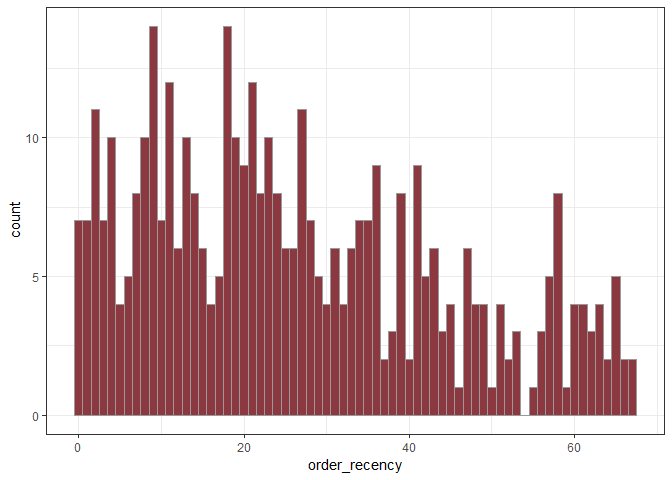ggplot(frm_tbl_initial, aes(x=order_frequency)) + geom_histogram(fill='#8b3840', color='grey60', binwidth = 1) + theme_bw()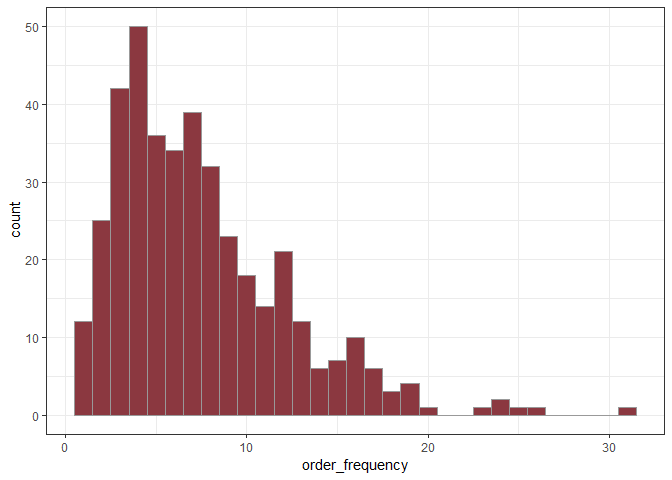Task: Demonstrate the code for the histogram of the monetary values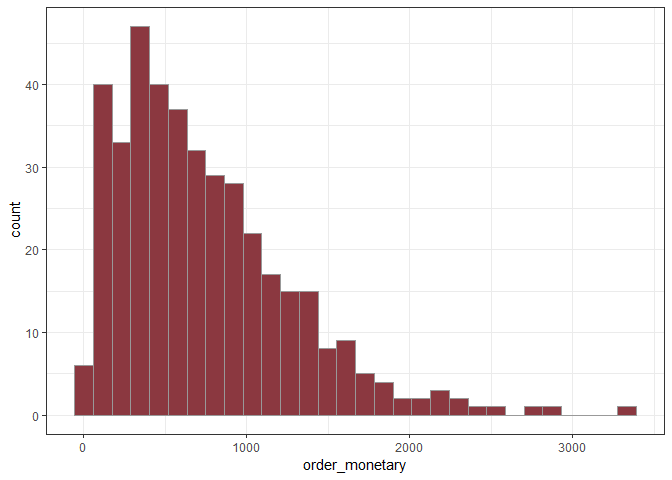Now, we need to define the limits of our RFM values and divide these intervals into bins. There are, again, many ways to proceed. We will use our domain knowledge and define cut points manually. However, we can define bins using quantiles, using equal intervals in terms of values or equal intervals in terms of number of observations in each bin.

Note. cut_interval makes n groups with equal range, cut_number makes n groups with (approximately) equal numbers of observations. cut function allows to specify cutting points manually.

summary(frm_tbl_initial) # to explore limits
##    client_id     order_frequency  order_recency   order_monetary
##  Min.   :  1.0   Min.   : 1.000   Min.   : 0.00   Min.   :   9.73
##  1st Qu.:136.0   1st Qu.: 4.000   1st Qu.:11.00   1st Qu.: 329.91
##  Median :286.0   Median : 7.000   Median :23.00   Median : 622.17
##  Mean   :281.7   Mean   : 7.491   Mean   :26.55   Mean   : 738.76
##  3rd Qu.:426.0   3rd Qu.:10.000   3rd Qu.:39.00   3rd Qu.:1008.34
##  Max.   :560.0   Max.   :31.000   Max.   :67.00   Max.   :3344.72

Here we set breaks according to explored data above:

fr_tbl <- mutate(frm_tbl_initial,
frequency_bins = cut(order_frequency,
breaks = c(0,4,7,8,10,31)))
table(fr_tbl$frequency_bins) ## ## (0,4] (4,7] (7,8] (8,10] (10,31] ## 129 109 32 41 90 fr_tbl <- mutate(fr_tbl, recency_bins = cut(order_recency, breaks = c(-1,11,23,26,39,67))) table(fr_tbl$recency_bins)
##
## (-1,11] (11,23] (23,26] (26,39] (39,67]
##     102     102      20      79      98
fr_tbl <- mutate(fr_tbl,
monetary_bins = cut(order_monetary,
breaks=c(9,330,622,739,1008,3345)))
table(fr_tbl$monetary_bins) ## ## (9,330] (330,622] (622,739] ## 101 99 34 ## (739,1.01e+03] (1.01e+03,3.34e+03] ## 66 101 Now, we have the clients allocated into bins, which provides already a good intution, what kind of customers and how many of those we have. We can explore 2-way and 3-way frequency tables: table(frequency=fr_tbl$frequency_bins, recency=fr_tbl$recency_bins) ## recency ## frequency (-1,11] (11,23] (23,26] (26,39] (39,67] ## (0,4] 22 26 6 32 43 ## (4,7] 26 29 6 14 34 ## (7,8] 6 9 2 12 3 ## (8,10] 8 11 2 9 11 ## (10,31] 40 27 4 12 7 table(frequency=fr_tbl$frequency_bins, recency=fr_tbl$recency_bins, monetary=fr_tbl$monetary_bins)
## , , monetary = (9,330]
##
##          recency
## frequency (-1,11] (11,23] (23,26] (26,39] (39,67]
##   (0,4]        14      17       3      18      30
##   (4,7]         6       3       2       2       6
##   (7,8]         0       0       0       0       0
##   (8,10]        0       0       0       0       0
##   (10,31]       0       0       0       0       0
##
## , , monetary = (330,622]
##
##          recency
## frequency (-1,11] (11,23] (23,26] (26,39] (39,67]
##   (0,4]         6       6       2      13       8
##   (4,7]        11      15       3       8      12
##   (7,8]         3       2       0       3       1
##   (8,10]        2       2       0       0       1
##   (10,31]       0       0       0       1       0
##
## , , monetary = (622,739]
##
##          recency
## frequency (-1,11] (11,23] (23,26] (26,39] (39,67]
##   (0,4]         1       3       0       1       1
##   (4,7]         2       7       1       2       6
##   (7,8]         0       1       0       0       1
##   (8,10]        1       1       0       2       2
##   (10,31]       0       0       1       1       0
##
## , , monetary = (739,1.01e+03]
##
##          recency
## frequency (-1,11] (11,23] (23,26] (26,39] (39,67]
##   (0,4]         1       0       1       0       3
##   (4,7]         5       3       0       2       8
##   (7,8]         1       4       0       4       1
##   (8,10]        2       4       1       5       4
##   (10,31]       7       9       0       0       1
##
## , , monetary = (1.01e+03,3.34e+03]
##
##          recency
## frequency (-1,11] (11,23] (23,26] (26,39] (39,67]
##   (0,4]         0       0       0       0       1
##   (4,7]         2       1       0       0       2
##   (7,8]         2       2       2       5       0
##   (8,10]        3       4       1       2       4
##   (10,31]      33      18       3      10       6

We can see from the table that the order of the bins is from the smallest value to the higher. However, if we want the best clients to be in the upper-left corner (also, later for the plots), we need to relevel our factor feature – bins. Recency can be the same - the more recent the purchase is, the better. However, for monetary and frequency scores we want it to be the opposite – the higher the bin, the better.

fr_tbl$frequency_bins <- factor(fr_tbl$frequency_bins, levels=rev(levels(fr_tbl$frequency_bins))) fr_tbl$monetary_bins <- factor(fr_tbl$monetary_bins, levels=rev(levels(fr_tbl$monetary_bins)))

table(frequency=fr_tbl$frequency_bins, recency=fr_tbl$recency_bins)
##          recency
## frequency (-1,11] (11,23] (23,26] (26,39] (39,67]
##   (10,31]      40      27       4      12       7
##   (8,10]        8      11       2       9      11
##   (7,8]         6       9       2      12       3
##   (4,7]        26      29       6      14      34
##   (0,4]        22      26       6      32      43

There are also some plots that may help us to understand our rfm tables better:

fr_tbl_counts <- fr_tbl %>% group_by(frequency_bins, recency_bins) %>% summarise(count=n())
p_basic <- ggplot(fr_tbl_counts, aes(x=recency_bins, y=frequency_bins)) + geom_tile(aes(fill=count))+
geom_text(aes(label = count)) +
p_basic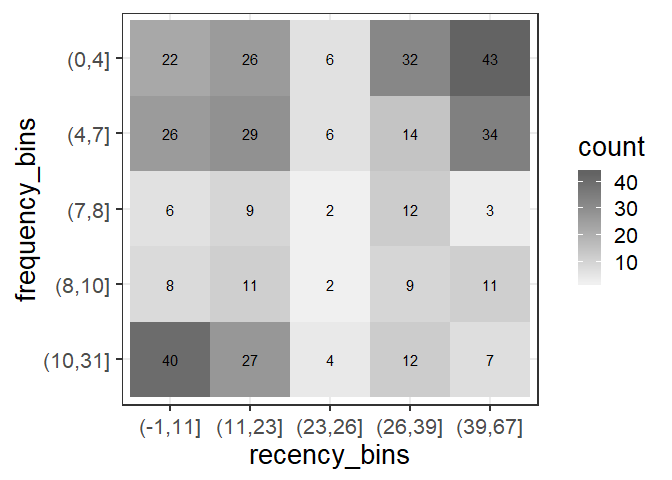p_quadrants <- p_basic +
ggplot2::annotate("rect", xmin = 0, xmax=3.47, ymin=3.47, ymax=6, color='green', alpha=0.1, fill='green') +
ggplot2::annotate("rect", xmin = 0, xmax=3.47, ymin=2.5, ymax=3.47, color='yellow', alpha=0.1, fill='yellow') +
ggplot2::annotate("rect", xmin = 0, xmax=3.47, ymin=0, ymax=2.5, color='blue', alpha=0.1, fill='blue') +
ggplot2::annotate("rect", xmin = 3.5, xmax=6, ymin=3.47, ymax=6, color='red', alpha=0.1, fill='red') +
ggplot2::annotate("rect", xmin = 3.5, xmax=6, ymin=0, ymax=3.47, color='black', alpha=0.1, fill='black')
p_quadrants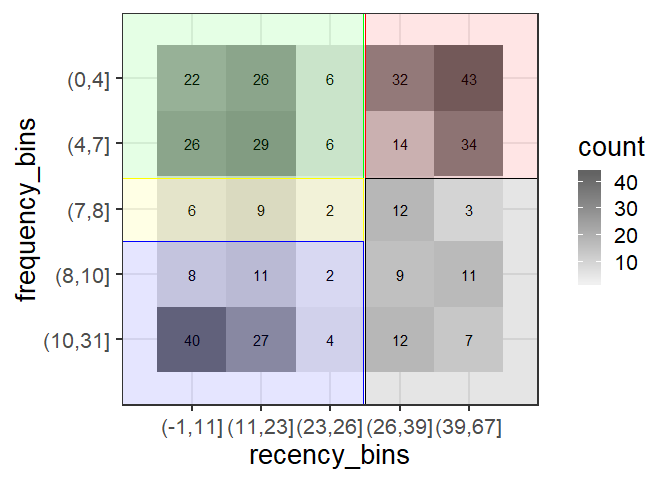p_quadrants +
ggplot2::annotate("text", x=1.8, y=5.8, label='New') +
ggplot2::annotate("text", x=4.8, y=5.8, label='Lost') +
ggplot2::annotate("text", x=1.4, y=2.7, label='Promising') +
ggplot2::annotate("text", x=1.8, y=0.2, label='Loyal customers') +
ggplot2::annotate("text", x=4.8, y=0.2, label='Hibernating loyal customers')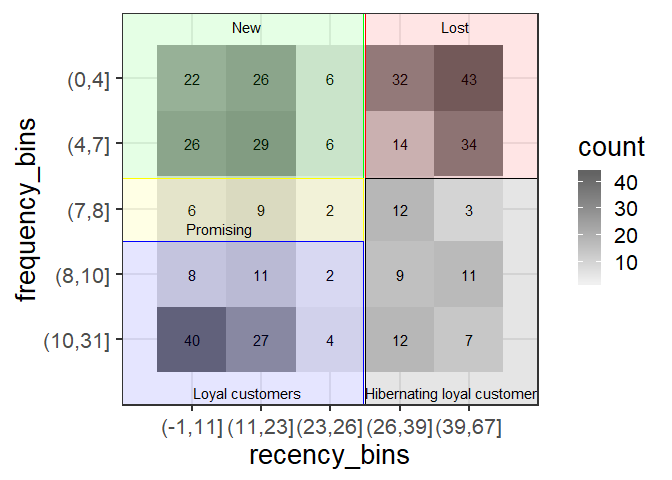Another approach to RFM calculation is to replace bins with numeric values, usually from 1 to 5, and create an RFM score which is just putting the numbers together. For example, a customer, who belongs to the first bin in R, to the second in F and to the fifth in M will have an RFM score of 125:

fr_tbl_score <- fr_tbl %>%
mutate(f_score = as.numeric(frequency_bins),
r_score = as.numeric(recency_bins),
m_score = as.numeric(monetary_bins)) %>%
mutate(RFM_score = paste(f_score, r_score, m_score, sep=''))
fr_tbl_score %>% arrange(RFM_score) %>% View
# will open a table in the view format, arrange sorts the data

The RFM approach is widely used and has a lot of use cases on practice. We can find our best and worst customers, we can decide where to focus our attention, to whom send the campaign and offer discounts. It is clear and reasonably easy to interpret (the only problem is how to visualize the three dimensions simultaniously). However, it requires your attention and highly depends on your choices. It is unlikely you can discover something unexpected. The automatic segmentation is a foray into uncharted territory of your data. Let’s see how it can be done.

## K-means clustering

Firstly, if we want to use k-means approach we need to scale features by substructing mean and dividing by standard deviation. This can be done using scale function. We firstly will cluster the previous dataset using only intial values (not scores or bins!)

data_clustering <- frm_tbl_initial %>%
mutate(order_frequency=scale(order_frequency),
order_recency=scale(order_recency),
order_monetary=scale(order_monetary))
head(data_clustering)
## # A tibble: 6 x 4
##   client_id order_frequency order_recency order_monetary
##       <int>           <dbl>         <dbl>          <dbl>
## 1         1          -0.313        -1.20         -0.315
## 2         2          -0.103        -1.36         -0.376
## 3         3          -0.103        -0.530         0.0555
## 4         4          -0.523        -1.14          1.10
## 5         5          -1.15          1.97         -1.33
## 6         6           0.107        -0.252        -0.423

Then, it is wise to explore what parameters kmeans method requires:

?kmeans
clusters <- kmeans(data_clustering[,-1], centers = 4, nstart=20)
clusters
## K-means clustering with 4 clusters of sizes 113, 139, 122, 27
##
## Cluster means:
##   order_frequency order_recency order_monetary
## 1       0.7233487    -0.3947948      0.7345606
## 2      -0.5635881    -0.6252380     -0.6036955
## 3      -0.5572271     1.2084793     -0.5102536
## 4       2.3919278    -0.5894290      2.3392321
##
## Clustering vector:
##    2 2 2 1 3 2 2 2 2 2 3 3 4 3 2 4 2 1 4 2 3 1 3 3 1 1 3 3 3 3 2 2 1 4 2
##   1 1 3 2 3 1 3 3 2 2 2 3 2 1 2 2 3 2 3 1 3 2 1 3 3 3 2 2 2 4 2 2 2 2 2
##   2 3 3 1 1 3 1 4 2 3 1 1 2 3 2 3 1 3 3 1 1 1 3 1 1 1 2 4 1 2 2 1 3 3 2
##  1 2 2 4 1 3 1 2 1 3 2 3 2 4 2 1 3 2 2 2 2 2 2 2 2 1 2 1 3 1 2 1 1 2 3
##  3 1 2 1 2 2 4 1 1 4 3 3 1 3 1 2 1 3 1 3 3 2 2 1 1 2 2 1 2 3 3 3 1 2 2
##  1 1 1 1 2 2 3 2 2 2 2 1 3 1 1 3 1 3 4 1 2 1 1 2 3 4 2 1 1 3 3 3 2 3 1
##  1 3 1 3 3 2 3 3 1 2 2 2 2 2 2 2 1 2 2 1 3 4 2 1 3 3 1 4 3 2 2 3 2 2 1
##  2 3 3 3 2 2 1 3 2 4 2 2 1 1 1 1 2 1 2 3 1 1 1 2 2 1 2 3 2 1 1 1 1 1 1
##  1 1 3 2 2 2 2 1 2 2 2 3 3 4 1 3 3 1 2 1 3 2 3 3 1 3 2 2 4 3 3 2 3 1 2
##  3 3 1 3 1 3 2 3 2 1 3 3 3 3 1 2 3 2 3 3 3 3 3 1 2 1 4 2 4 3 2 4 2 3 2
##  2 2 4 2 3 1 3 3 2 2 1 4 3 1 2 1 1 3 2 1 3 3 4 3 1 2 3 3 3 3 1 3 3 2 3
##  1 1 2 1 3 2 4 3 3 4 1 4 2 1 2 3
##
## Within cluster sum of squares by cluster:
##  105.15080  77.53735 106.51189  64.52544
##  (between_SS / total_SS =  70.5 %)
##
## Available components:
##
##  "cluster"      "centers"      "totss"        "withinss"
##  "tot.withinss" "betweenss"    "size"         "iter"
##  "ifault"

Let us assgn clusters to the clients, by adding it to the data frame

data_clustering$cluster <- as.factor(clusters$cluster) # we need it to be a factor for the plot
ggplot(data_clustering, aes(x=order_frequency, y=order_recency, color=cluster)) + geom_point(size=2) + theme_bw()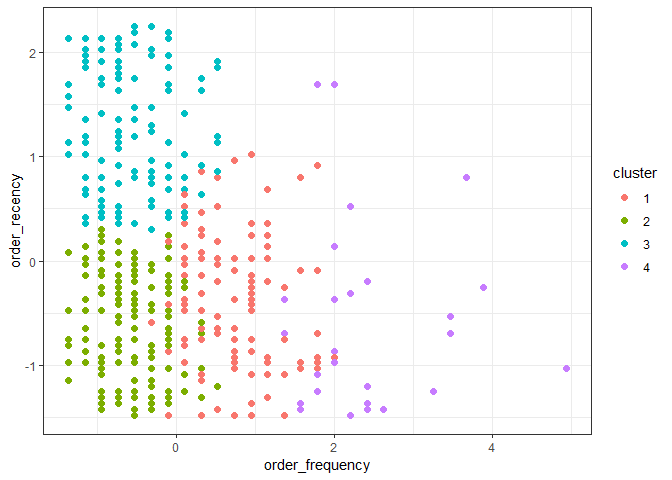We can plot all three plots together:

#gridExtra
p1 <- ggplot(data_clustering, aes(x=order_frequency, y=order_recency, color=cluster)) + geom_point(size=2) + theme_bw()
p2 <- ggplot(data_clustering, aes(x=order_frequency, y=order_monetary, color=cluster)) + geom_point(size=2) + theme_bw()
p3 <- ggplot(data_clustering, aes(x=order_monetary, y=order_recency, color=cluster)) + geom_point(size=2) + theme_bw()
grid.arrange(p1,p2,p3, nrow=3)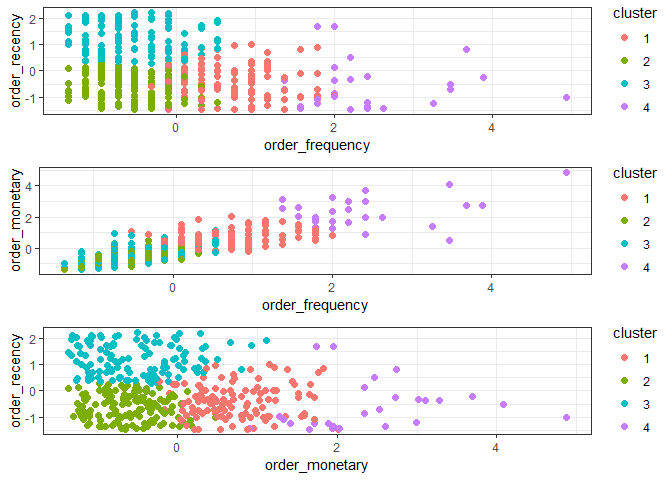The reasonable question to ask is how to choose number of clusters? It is usually the iterative process where the clusters should be manually inspected. You should be asking the following questions. Are they are different enough? Do they make sense? If I change number of clusters, does the structure changes a lot or the pattern of clusters persists? There are also various techniques how to identify number of clusters. These methods provide you with some additional intuition (but they do not guarantee this is the best number of clusters for your problem). Also, be aware that the clusters can be found even when the underlying structure does not have them. We will take a look at the most common elbow method.

# finding optimal number of clusters
elbow_method <- function(data, max_k=15){
require(ggplot2)
wss <- 0
for (i in 1:max_k){
wss[i] <- sum(kmeans(data, centers=i)$withinss) } p <- data.frame(number_of_clusters=c(1:max_k), wss=wss) %>% ggplot(aes(x=number_of_clusters, y=wss)) + geom_point() + geom_line() + theme_bw() + ylab("Within groups sum of squares") return(print(p)) } # apply the function elbow_method(data_clustering[,-1], max_k=15)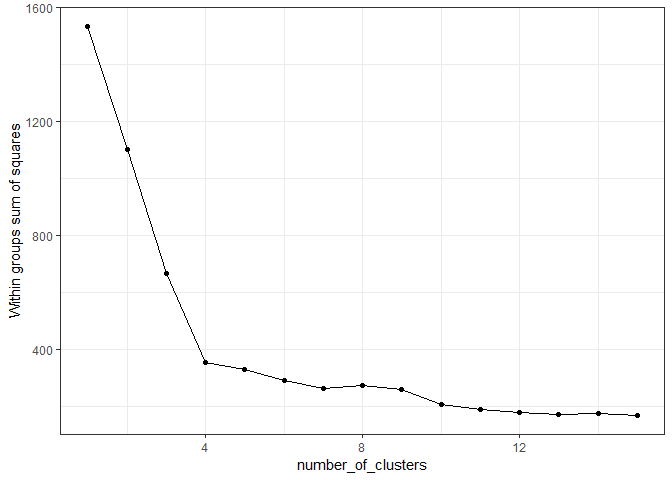Usually there is a tradeoff, we want as few clusters as possible, because it is easy to interpret them (nobody wants to interpret and act upon 79 segments of customers), but we want to minimize the wss statistics. The idea behind the elbow methods is that we want to find a point, where the wss is small, but the function does not become smooth (adding more clusters does not reduce much of the variance), so we are looking for an angle in the curve. ## Real-life grocery dataset Currently we don’t know much about the customers themselves, which makes difficult to interpret how meaningful is the segmentation. In general, we shouldn’t limit ourselves to RFM values, we can combine many features. Also, not only customers can be segmented, we can cluster products, purchases, product baskets or even sequences of actions in time. Let’s take a look at the real-life large dataset. The details about it and the dataset itself can be found here: âThe Instacart Online Grocery Shopping Dataset 2017â. Let’s use a library data.table to speed up the process. dt_orders <- fread(file.choose()) # orders.csv dt_products <- fread(file.choose()) # order_products__prior.csv product_names <- fread(file.choose()) # products.csv First, we should examine the data: head(dt_orders,3) ## order_id user_id eval_set order_number order_dow order_hour_of_day ## 1: 2539329 1 prior 1 2 8 ## 2: 2398795 1 prior 2 3 7 ## 3: 473747 1 prior 3 3 12 ## days_since_prior_order ## 1: NA ## 2: 15 ## 3: 21 head(dt_products,3) ## order_id product_id add_to_cart_order reordered ## 1: 2 33120 1 1 ## 2: 2 28985 2 1 ## 3: 2 9327 3 0 head(product_names,3) ## product_id product_name aisle_id department_id ## 1: 1 Chocolate Sandwich Cookies 61 19 ## 2: 2 All-Seasons Salt 104 13 ## 3: 3 Robust Golden Unsweetened Oolong Tea 94 7 We need to merge product ids with their names and then orders and products by order_id: dt_products <- left_join(dt_products, product_names[,c(1,2)], by="product_id") head(dt_products,3) ## order_id product_id add_to_cart_order reordered product_name ## 1 2 33120 1 1 Organic Egg Whites ## 2 2 28985 2 1 Michigan Organic Kale ## 3 2 9327 3 0 Garlic Powder dt_full <- left_join(dt_orders, dt_products, by='order_id') head(dt_full,3) ## order_id user_id eval_set order_number order_dow order_hour_of_day ## 1 2539329 1 prior 1 2 8 ## 2 2539329 1 prior 1 2 8 ## 3 2539329 1 prior 1 2 8 ## days_since_prior_order product_id add_to_cart_order reordered ## 1 NA 196 1 0 ## 2 NA 14084 2 0 ## 3 NA 12427 3 0 ## product_name ## 1 Soda ## 2 Organic Unsweetened Vanilla Almond Milk ## 3 Original Beef Jerky Let’s now define what features we want to use for the clustering of products. Note. Don’t forget to take a look at the dictionary that explains feature names: dictionary The list of the features that we want to extract: • how many times each product was bought • how many unique users bought it • in how many different orders this product appears • most frequent day of week • most frequent hour of the day • what is the average number of day since the last order • most frequent order of adding it to the cart • how frequently it was bought before For my convinience I specify the most frequent function in advance, which is the Mode statistic (returns the most frequent element): freq_value <- function(x) { ux <- unique(x) ux[which.max( tabulate(match(x, ux)) # number of times each item occurs )] } Feature collection: features <- dt_full %>% group_by(product_id) %>% # we want all this calculated per product summarise(count=n(), # total orders unique_users=length(unique(user_id)), # number of unique users unique_orders=length(unique(order_id)), freq_ord_dow = freq_value(order_dow), # the most frequent day of the week freq_hour=freq_value(order_hour_of_day), avg_days_prior=mean(days_since_prior_order,na.rm=TRUE), # av. of days since last order freq_add_to_cart = freq_value(add_to_cart_order), # the most frequent order in which product was added reordered_n = sum(reordered)) # amount of reorders Note that na.rm=TRUE. If you check the summary, you discover that we have missing values. If we do not highlight that for average claculaton we ignore missing values, the result will be NA. Apparently, for r (3+2+NA)/3 = NA. ## Missing data problem So, how to work with missing data. It is a very broad topic itself. Let’s see how we can deal with it: filter(features, is.na(product_id)==TRUE) # always observe your missing values before proceeding!  ## # A tibble: 1 x 9 ## product_id count unique_users unique_orders freq_ord_dow freq_hour ## <int> <int> <int> <int> <int> <int> ## 1 NA 206209 206209 206209 0 15 ## # ... with 3 more variables: avg_days_prior <dbl>, freq_add_to_cart <int>, ## # reordered_n <int> features <- filter(features, is.na(product_id)==FALSE) We can’t do anything with the product_id being missing as it is id. We remove it. Now, we proceed the same as previously, we scale our features: #dplyr scaled_features <- mutate_at(features, vars(-product_id), scale) scaled_features ## # A tibble: 49,677 x 9 ## product_id count unique_users unique_orders freq_ord_dow freq_hour ## <int> <dbl> <dbl> <dbl> <dbl> <dbl> ## 1 1 0.250 0.342 0.250 -0.479 -1.03 ## 2 2 -0.117 -0.145 -0.117 1.80 -0.690 ## 3 3 -0.0784 -0.148 -0.0784 -0.479 -0.690 ## 4 4 -0.0676 -0.0656 -0.0676 -0.935 0.328 ## 5 5 -0.133 -0.200 -0.133 -0.935 -0.350 ## 6 6 -0.135 -0.201 -0.135 1.80 0.667 ## 7 7 -0.130 -0.191 -0.130 0.433 0.667 ## 8 8 -0.102 -0.142 -0.102 -0.935 -0.0112 ## 9 9 -0.104 -0.148 -0.104 1.80 -0.0112 ## 10 10 0.400 0.764 0.400 -0.479 -0.690 ## # ... with 49,667 more rows, and 3 more variables: avg_days_prior <dbl>, ## # freq_add_to_cart <dbl>, reordered_n <dbl> However, we still have missing values and cannot run kmeans. Why? Let us take a look: filter(features, is.na(avg_days_prior)==TRUE) %>% View filter(dt_full, product_id==4908) # product appears only once and average days are missing ## order_id user_id eval_set order_number order_dow order_hour_of_day ## 1 1178215 185887 prior 1 3 18 ## days_since_prior_order product_id add_to_cart_order reordered ## 1 NA 4908 10 0 ## product_name ## 1 Smooth & Silky Head and Shoulders Smooth & Silky 2-in-1 Dandruff Shampoo + Conditioner 13.5 Fl Oz Female Hair Care We can see that only average_days_prior have missing values. scaled_features <- na.omit(scaled_features) Let’s find amount of clusters first: elbow_method(data=scaled_features, max_k=30)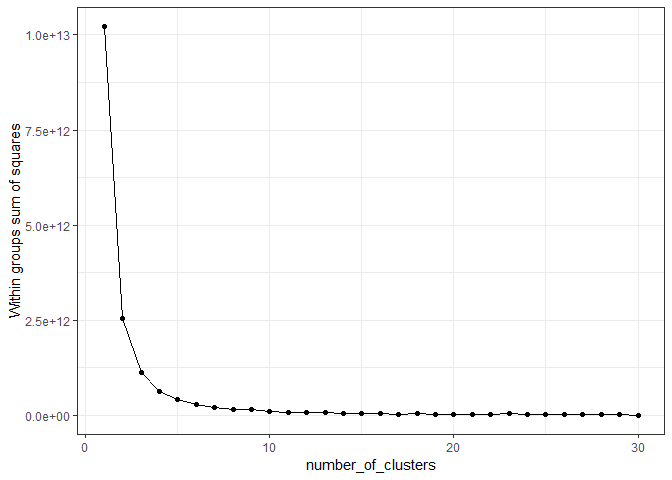clusters <- kmeans(scaled_features, centers = 4, nstart=20) features_with_cluster <- na.omit(features) features_with_cluster$cluster <- as.factor(clusters$cluster) features_with_cluster <- left_join(features_with_cluster, product_names[,c(1,2)], by="product_id") # to see what are the product names Let’s visualize it. ggplot(features_with_cluster, aes(x=count, y=unique_users, color=cluster)) + geom_point() + theme_bw() + scale_x_log10() + scale_y_log10()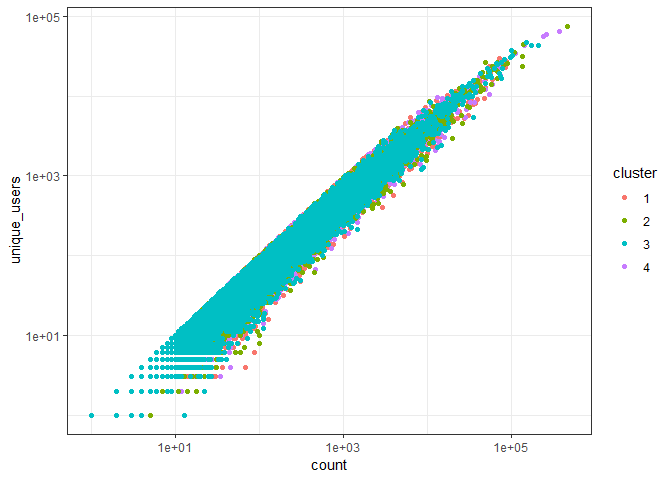ggplot(features_with_cluster, aes(x=freq_ord_dow)) + geom_density() + facet_grid(~cluster)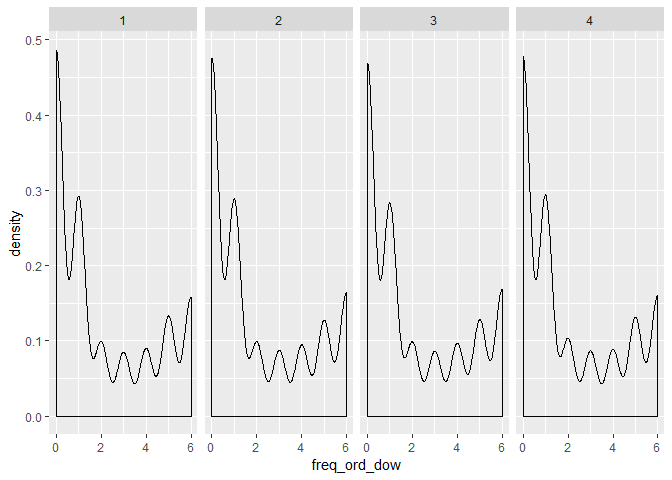Now let’s use another ways of visualization corpus <- Corpus(VectorSource( filter(features_with_cluster, cluster==2)$product_name))
tdm <- TermDocumentMatrix(corpus)
m <- as.matrix(tdm)
v <- sort(rowSums(m),decreasing=TRUE)
d <- data.frame(word = names(v),freq=v)
wordcloud(d$word,d$freq, min.freq=2, max.words=50)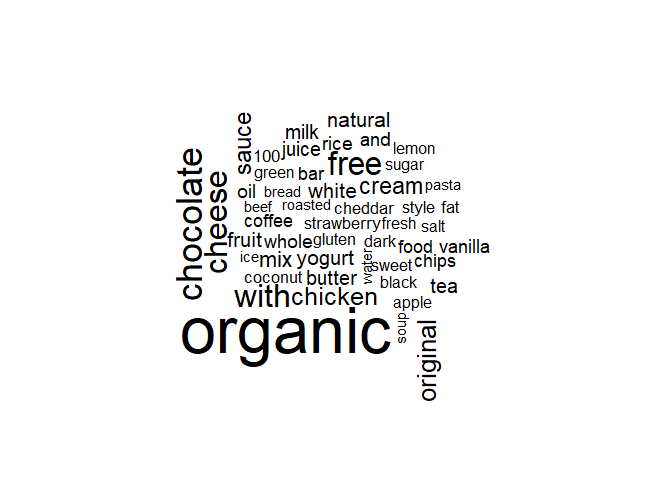You can change the cluster number and get the results of other clusters. By changing min.freq and max.words you can regulate the thresholds for word frequency to display and the amount of words to show.

## Hierarchical clustering

The second clustering method that we can use for segmentation is hierarchical clustering

distance_m <- dist(as.matrix(frm_tbl_initial[,-1]))
hc <- hclust(distance_m)
plot(hc)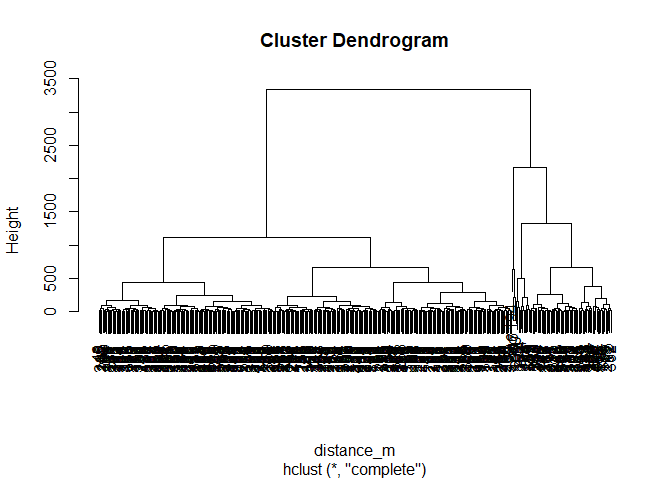Let’s take a small set of the data:

#small sample of data
features <- left_join(features, product_names[,c(1,2)], by="product_id")
features_sample <- sample_n(features, size = 100)
features_sample <- as.data.frame(features_sample) # we need this function just because the dendrogram cannot see labels
row.names(features_sample) = features_sample$product_name # we will assign product names as row names. just strange unlogical behaviour of dendogram plot features_sample$product_name <- NULL
distance_m <- dist(as.matrix(features_sample[,-c(1)]))
hc <- hclust(distance_m)
plot(hc)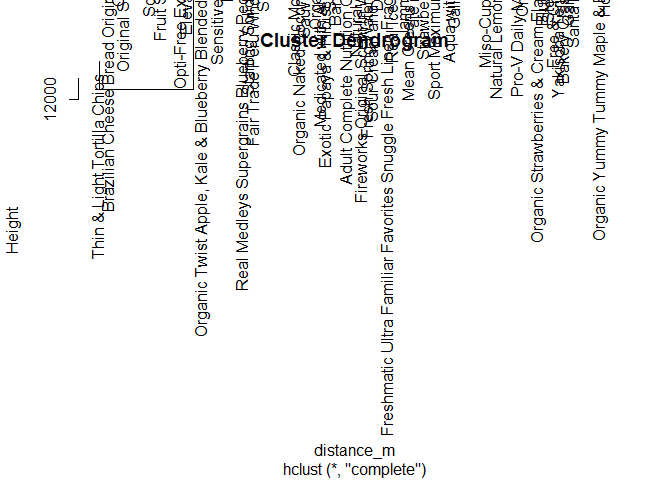A few other ways to plot a dendrogram (don’t forget to install packages first):

plot(as.phylo(hc), type = "fan")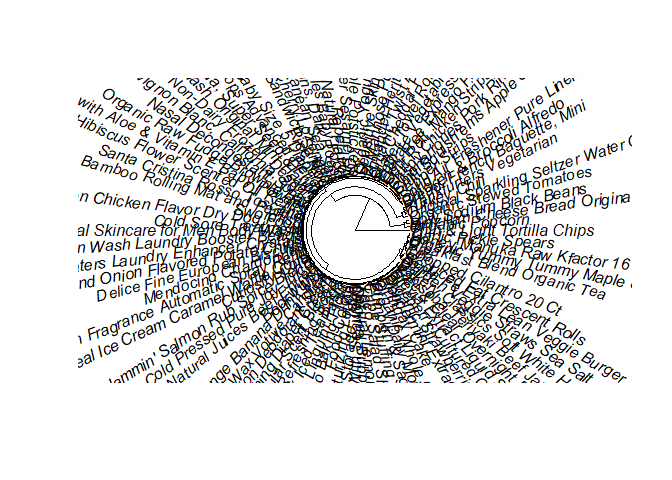ggdendrogram(hc, rotate = TRUE, size = 4)